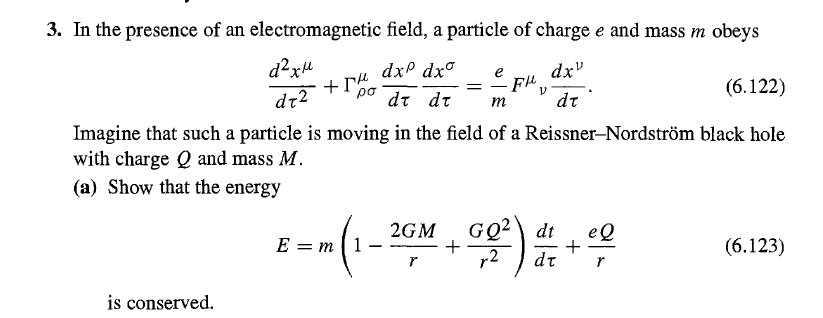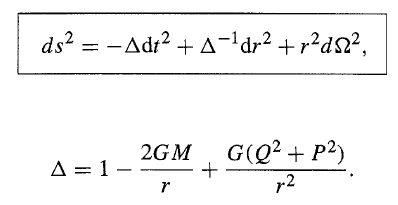# Show that the energy is conserved in this field/metric

LCSphysicist
Homework Statement:
...
Relevant Equations:
...I would like it very much if someone could give a hint on how to start this question.

In particular, I tried to find the derivative of energy with respect to time, but that was not enough.
Then I tried to apply the formula p * p = -m², but that also didn't get me anywhere.
These were my two attempts, I imagine there is another way but I haven't been able to find it yet

This is the metric:The "magnetic charge" P is zero, at least i think so.

I thought in another way, try to find any Killing vector and see its relation with the energy, but i am not sure if this is will be helpful

•PeroK

Homework Helper
Gold Member
2022 Award

•Mentor
2022 Award
Moved.

Homework Helper
Gold Member
Homework Statement:: ...
View attachment 279056
This problem is from Sean Carroll's textbook.
I thought in another way, try to find any Killing vector and see its relation with the energy, but i am not sure if this is will be helpful.
Yes, there is a Killing vector or isometry that is relevant to this problem.

Review section 3.8 on symmetries and Killing vectors. Equations (3.161), (3.162), (3.167) and (3.168) in this section are for particles following geodesics. How would these equations be modified if the geodesic equation [(3.44) or (3.161)] is replaced by equation (6.122) in the problem statement?